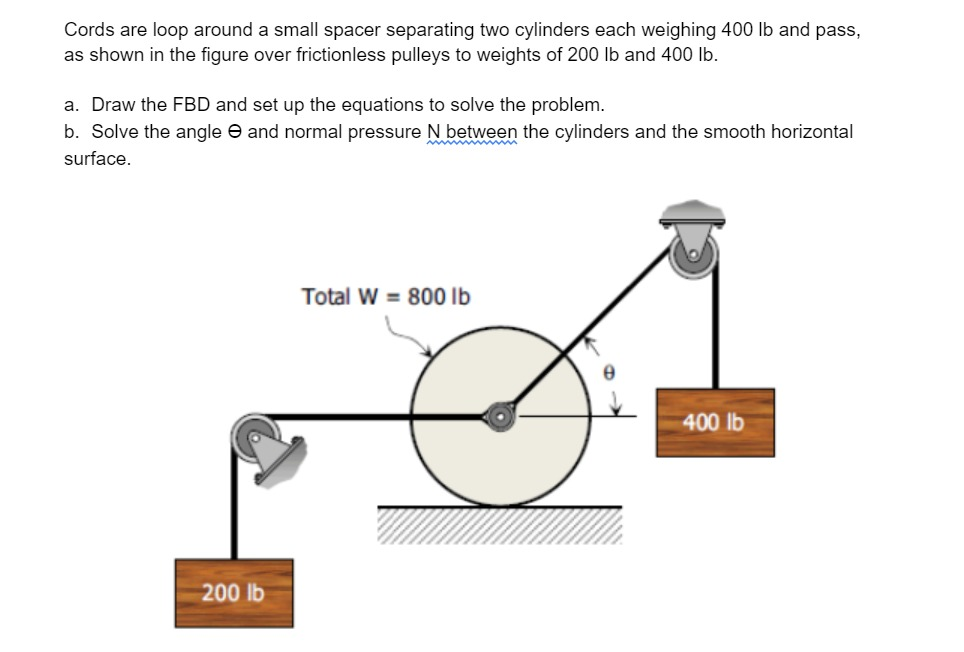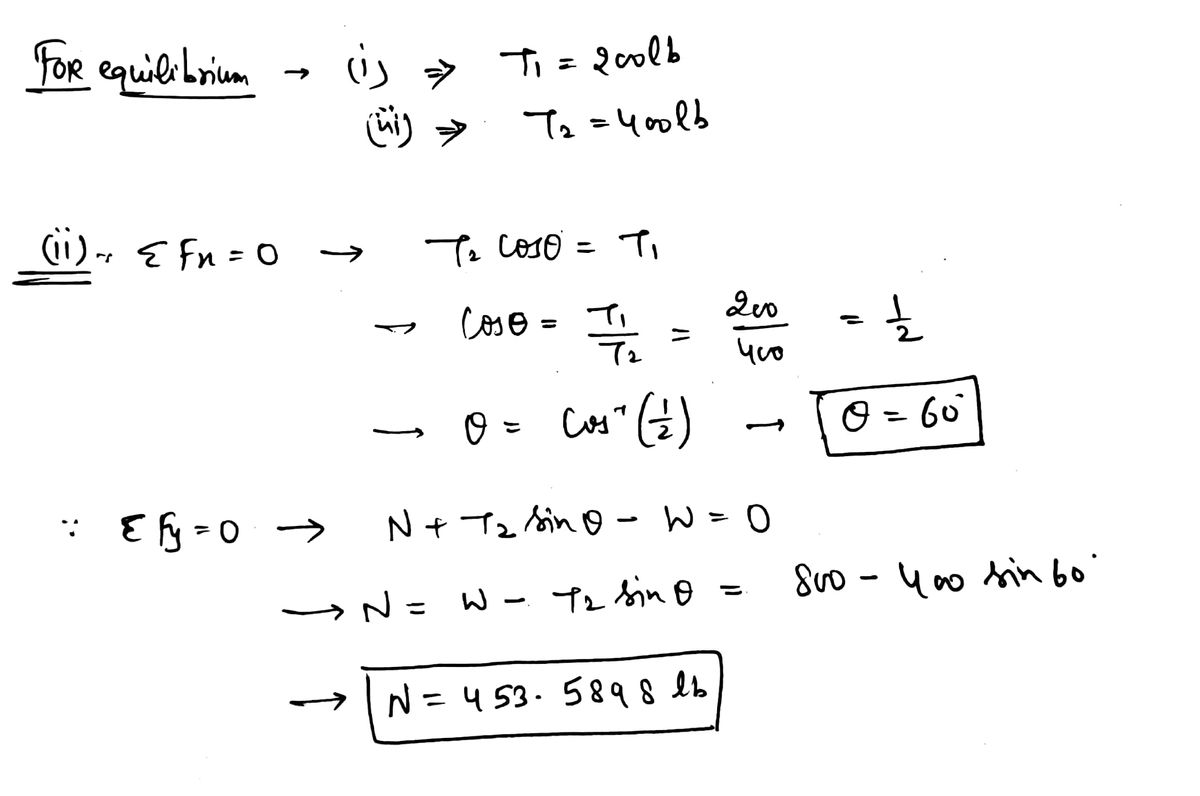# Cords are loop around a small spacer separating two cylinders each weighing 400 lb and pass, as shown in the figure over frictionless pulleys to weights of 200 lb and 400 Ib. a. Draw the FBD and set up the equations to solve the problem. b. Solve the angle e and normal pressure N between the cylinders and the smooth horizontal surface.

Question-AnswerCategory: Engineering MechanicsCords are loop around a small spacer separating two cylinders each weighing 400 lb and pass, as shown in the figure over frictionless pulleys to weights of 200 lb and 400 Ib. a. Draw the FBD and set up the equations to solve the problem. b. Solve the angle e and normal pressure N between the cylinders and the smooth horizontal surface.

### Cords are loop around a small spacer separating two cylinders each weighing 400 lb and pass, as shown in the figure over frictionless pulleys to weights of 200 lb and 400 Ib. a. Draw the FBD and set up the equations to solve the problem. b. Solve the angle e and normal pressure N between the cylinders and the smooth horizontal surface.